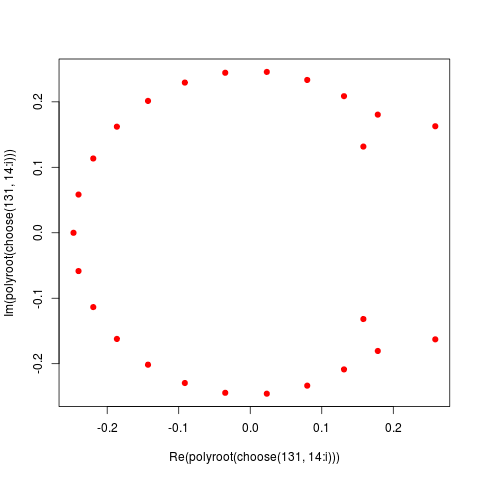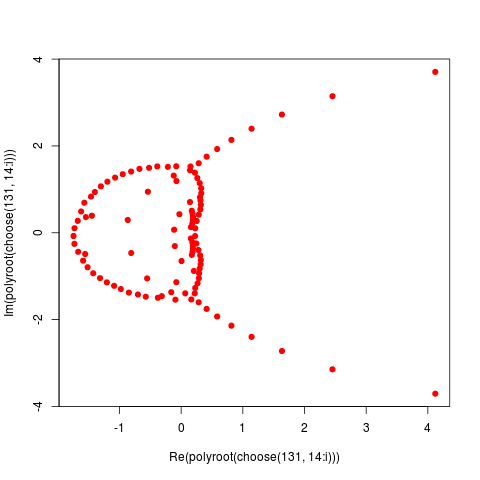Want to share your content on R-bloggers? click here if you have a blog, or here if you don't.2939597999119“139”

```> plot( polyroot(choose(131,14:29)) ,pch=19,col='red')
> plot( polyroot(choose(131,14:39)) ,pch=19,col='red')
> plot( polyroot(choose(131,14:59)) ,pch=19,col='red')
> plot( polyroot(choose(131,14:79)) ,pch=19,col='red')
> plot( polyroot(choose(131,14:99)) ,pch=19,col='red')
> plot( polyroot(choose(131,14:119)) ,pch=19,col='red')
> plot( polyroot(choose(131,14:139)) ,pch=19,col='red')
```